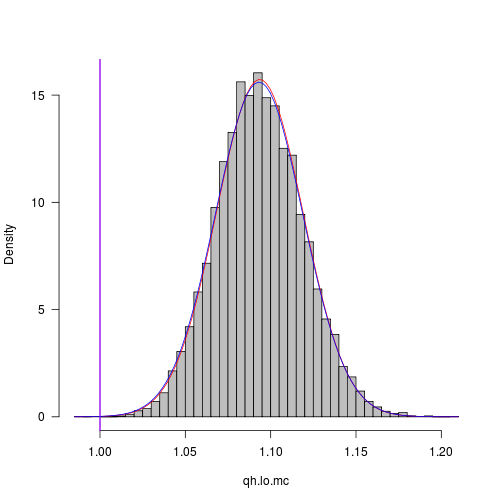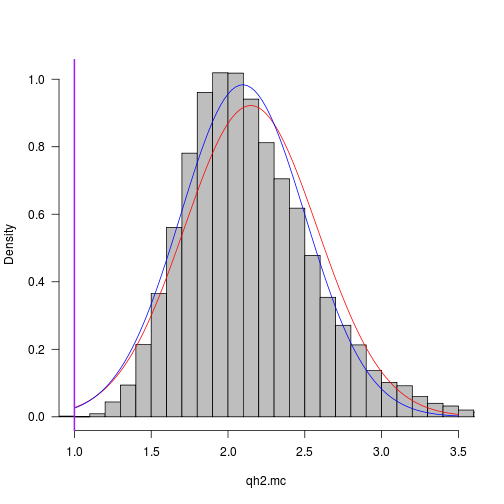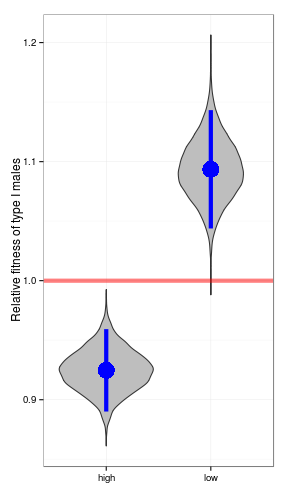# Uncertainty of fitness ratios

Version: 2012-12-19 20:03:40

From Karen C.:

q.mean = 0.915
q.var = 0.00014
h.lo.mean = 0.837
h.lo.var = 0.000266
h.hi.mean = 0.9897
h.hi.var = 0.000199


Delta method by hand (i.e. using $\mbox{CV}^2(q/h) = \mbox{CV}^2(q)+\mbox{CV}^2(h) \\ \mbox{Var}(q/h)/(q^2/h^2) = \mbox{Var}(q)/q^2 + \mbox{Var}(h)/h^2 \\ \mbox{Var}(q/h) = 1/h^2 \mbox{Var}(q) + q^2/h^4 \mbox{Var}(h)$

(qh.lo.mean <- q.mean/h.lo.mean)

##  1.093

(qh.lo.var <- q.var/(h.lo.mean)^2 + h.lo.var * q.mean^2/h.lo.mean^4)

##  0.0006536

(qh.lo.se <- sqrt(qh.lo.var))

##  0.02557


Using an automated delta-variance calculator from the emdbook package:

library(emdbook)
deltavar(q/h, mean = c(q = q.mean, h = h.lo.mean), Sigma = c(q.var, h.lo.var))

##  0.0006536


Confidence limits:

(qh.lo.ci1 <- qh.lo.mean + c(-1, 1) * 1.96 * qh.lo.se)

##  1.043 1.143


Monte Carlo approach: pick many random normal deviates for $$q$$ and for $$h$$ and calculate their ratio:

mcsim_N <- 10000
set.seed(101)
q.mc <- rnorm(mcsim_N, q.mean, sqrt(q.var))
h.lo.mc <- rnorm(mcsim_N, h.lo.mean, sqrt(h.lo.var))
qh.lo.mc <- q.mc/h.lo.mc
par(las = 1, bty = "l")
hist(qh.lo.mc, col = "gray", breaks = 50, main = "", freq = FALSE)
curve(dnorm(x, mean = mean(qh.lo.mc), sd = sd(qh.lo.mc)), add = TRUE, col = 2)
abline(v = 1, col = "purple", lwd = 2)
curve(dnorm(x, mean = qh.lo.mean, sd = qh.lo.se), add = TRUE, col = 4)sd(qh.lo.mc)  ## estimated std err from MC

##  0.02536

quantile(qh.lo.mc, c(0.025, 0.975))  ## Monte Carlo confidence intervals

##  2.5% 97.5%
## 1.045 1.145


(The red line is the normal approximation to the MC distribution: the blue line is the distribution assumed/estimated by the delta method.) This indicates that the delta method is not too bad …

MC for high case:

h.hi.mc <- rnorm(mcsim_N, h.hi.mean, sqrt(h.hi.var))
qh.hi.mc <- q.mc/h.hi.mc


If you want do this for $$q/(1-q) \cdot (1-h)/h$$, it will be easiest to use the automated deltavar calculation or the MC approach (I could figure out the equation by hand but I don't want to bother), e.g.

qh2.mean <- q.mean/h.lo.mean * (1 - h.lo.mean)/(1 - q.mean)
qh2.mc <- q.mc/h.lo.mc * (1 - h.lo.mc)/(1 - q.mc)
quantile(qh2.mc, c(0.025, 0.975))

##  2.5% 97.5%
## 1.461 3.151

qh2.dv <- deltavar(q/h * (1 - h)/(1 - q), mean = c(q = q.mean, h = h.lo.mean),
Sigma = c(q.var, h.lo.var))
qh2.se <- sqrt(qh2.dv)
par(las = 1, bty = "l")
hist(qh2.mc, col = "gray", breaks = 50, main = "", freq = FALSE, xlim = c(1,
3.5))
curve(dnorm(x, mean = mean(qh2.mc), sd = sd(qh2.mc)), add = TRUE, col = 2)
curve(dnorm(x, mean = qh2.mean, sd = qh2.se), add = TRUE, col = 4)
abline(v = 1, col = "purple", lwd = 2)library(ggplot2)
d <- data.frame(qh = c(qh.lo.mc, qh.hi.mc), v = rep(c("low", "high"), each = mcsim_N))
theme_set(theme_bw())
ff <- function(x) {
result <- data.frame(y = mean(x), ymin = mean(x) - 1.96 * sd(x), ymax = mean(x) +
1.96 * sd(x))
}
ggplot(d, aes(x = v, y = qh)) + geom_violin(fill = "gray") + geom_hline(yintercept = 1,
colour = "red", lwd = 2, alpha = 0.5) + stat_summary(fun.data = ff, colour = "blue",
size = 2) + labs(x = "", y = "Relative fitness of type I males")